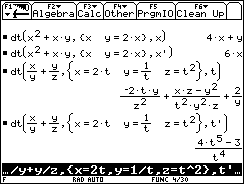## Texas Instruments TI-89 and TI-92 Plus Computer

Last page update: Sunday, November 21, 1999

 Total Derivative (Chain Rule) - for the TI-89 and TI-92 Plus Version 1.0   (Monday, September 06, 1999) Download [ dt.89f  | dt.9xf ] (883 bytes) Description Determines the total derivative of a multivariable function. Screen shotsDocumentation Usage:  dt(ContinuousFunc,{IndependantFunc1,IndependantFunc2,...},IndependantVar)  ContinuousFunc: A function of one or more functions or variables.  IndependantFunc: The variable or function definition. Do not enter x=x, y=y, etc., for any expression. If a variable does not represent an independant function then list only the variable (see x in example 2). If x=x (etc.) is used, it will evaluate to "true" and the function can not tell which variable is being used.  IndependantVar: The variable to differentiate with respect to. If this variable is postfixed with the prime symbol ('), the returned expression will be in terms of IndependantVar, otherwise it will be in terms of the IndependantFunc's (see examples). The prime symbol is [2nd][b] on the TI-92 and [2nd][=] on the TI-89.    Return value:  This function returns the total derivative, also known as the Chain Rule.  Examples:  1. If u=x/y+y/z, and if x=2t, y=1/t, z=t2, the total derivative of u is     a.  dt(x/y+y/z,{x=2t,y=1/t,z=t2},t) [in terms of the IndependantFunc's x, y, z]         =  -2ty/z2+(xz-y2)/(t2y2z)+2/y      b.  dt(x/y+y/z,{x=2t,y=1/t,z=t2},t') [in terms of the IndependantVar (t')]         =  (4t5-3)/t4      2. If u=x2+xy, and if y=2x, then      a.  dt(x2+xy,{x,y=2x},x) [in terms of the IndependantFunc's x, y]         = 4x+y      b.  dt(x2+xy,{x,y=2x},x') [in terms of the IndependantVar (x')]         = 6x